Download Presentation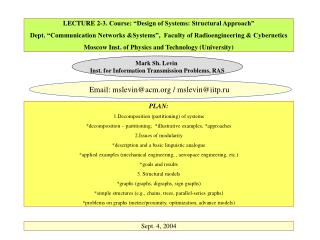LECTURE 2-3. Course: “Design of Systems: Structural Approach” Dept. “Communication Networks &Systems”, Faculty of

# LECTURE 2-3. Course: “Design of Systems: Structural Approach” Dept. “Communication Networks &Systems”, Faculty of - PowerPoint PPT Presentation

LECTURE 2-3. Course: “Design of Systems: Structural Approach” Dept. “Communication Networks &Systems”, Faculty of Radioengineering & Cybernetics Moscow Inst. of Physics and Technology (University) . Mark Sh. Levin Inst. for Information Transmission Problems, RAS.I am the owner, or an agent authorized to act on behalf of the owner, of the copyrighted work described.
Download Presentation## LECTURE 2-3. Course: “Design of Systems: Structural Approach” Dept. “Communication Networks &Systems”, Faculty of

An Image/Link below is provided (as is) to download presentation

Download Policy: Content on the Website is provided to you AS IS for your information and personal use and may not be sold / licensed / shared on other websites without getting consent from its author.While downloading, if for some reason you are not able to download a presentation, the publisher may have deleted the file from their server.

- - - - - - - - - - - - - - - - - - - - - - - - - - E N D - - - - - - - - - - - - - - - - - - - - - - - - - -
Presentation Transcript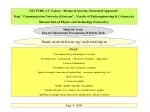Dept. “Communication Networks &Systems”, Faculty of Radioengineering & Cybernetics

Moscow Inst. of Physics and Technology (University)

Mark Sh. Levin

Inst. for Information Transmission Problems, RAS

Email: mslevin@acm.org / mslevin@iitp.ru

PLAN:

1.Decomposition (partitioning) of systems

*decomposition – partitioning; *illustrative examples; *approaches

2.Issues of modularity

*description and a basic linguistic analogue

*applied examples (mechanical engineering, , aerospace engineering, etc.)

*goals and results

3. Structural models

*graphs (graphs, digraphs, sign graphs)

*simple structures (e.g., chains, trees, parallel-series graphs)

*problems on graphs (metric/proximity, optimization, advance models)

Sept. 4, 2004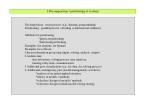1.Decomposition / partitioning of systems

Decomposition: series process (e.g., dynamic programming)

Partitioning: parallel process / dividing (combinatorial synthesis)

Methods for partitioning:

*physical partitioning

*functional partitioning

Examples (for airplane, for human)

Examples for software:

1.Series information processing (input, solving, analysis, output)

2.Architecture:

data subsystem, solving process, user interface,

training subsystem, communication

3.Additional part: visualization (e.g., for data, for solving process)

4. Additional contemporary part (model management) as follows:

*analysis of an initial applied situation,

*library of models / methods,

*selection / design of models / methods,

*selection / design of multi-model solving strategy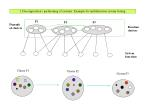1.Decomposition / partitioning of systems: Example for multifunction system testing

F1

F2

F3

Digraph

of clusters

Function

clusters

System

functions

Cluster F1

Cluster F2

Cluster F3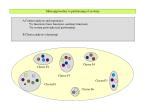Main approaches to partitioning of systems

A.Content analysis and experience:

*by functions (basic functions, auxiliary functions)

*by system parts (physical partitioning)

B.Cluster analysis (clustering)

Cluster F4

Cluster F2

Cluster F3

Cluster F5

Cluster F1

Cluster F6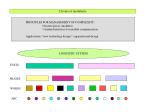2.Issues of modularity

PRINCIPLES FOR MANAGEMENT OF COMPLEXITY :

*discrete pieces (modules)

*standard interfaces for module communication

Applications: *new technology design * organizational design

LINGUISTIC SYSTEM

TEXTS

PRASES

WORDS

ABC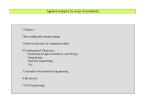Applied examples for usage of modularity

1.Genetics

2.Reconfigurable manufacturing

3.Software libraries of standard modules

4.Combinatorial Chemistry:

*drug design

*material engineering

*etc.

5.Aerospace & mechanical engineering

6.Electronics

7.Civil engineering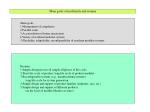Main goals of modularity and resume

Main goals:

1.Management of complexity

2.Parallel work

3.Accomodation of future uncertainty

4.Variety of resultant modular systems

5.Flexibility, adaptability, reconfigurability of resultant modular systems

Resume:

1.Simple design process & simple all phases of life cycle

2.Short life cycle of product, long life cycle of product modules

3.Reconfigurable systems (e.g., manufacturing systems):

long life cycle for system generation

4.Simple design and support of product families (airplanes, cars, etc.)

5.Simple design and support of different products

(on the basis of module libraries as reuse)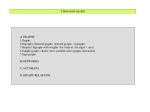3.Structural models

A.GRAPHS

1.Graphs

2.Digraphs (directed graphs, oriented graphs - orgraphs)

3.Graphs / digraphs with weights (for vertices, for edges / arcs)

4.Simple graphs: chains, trees, parallel-series graphs, hierarchies

5.Sign graphs

B.NETWORKS

C.AUTOMATA

D.BINARY RELATIONS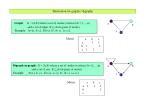Illustration for graphs / digraphs

Graph: G = (A,E) where a set of nodes (vertices) A={1,…,n}

and a set of edges E A×A (pairs of nodes)

Example: A={a, b, c}, E={(a, b), (b, c), (a, c)}

a

b

c

Matrix

a b c

a 1 1

b 1 1

c 1 1

Digraph (orgraph): G = (A,E) where a set of nodes (vertices) A={1,…,n}

and a set of arcs E A×A (pairs of nodes)

Example: A={a, b, c}, E={(a, a), (a, b), (b, c), (a, c)}

a

b

c

Matrix

a b c

a 1 1 1

b 1

c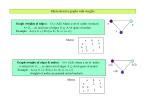Illustration for graphs with weights

Graph (weights of edges): G = (A,E) where a set of nodes (vertices)

A={1,…,n} and a set of edges E  A×A (pairs of nodes)

Example: A={a, b, c}, E={(a, b), (b, c), (a, c)}

a

b

c

Matrix

a b c

a 2 5

b 2 3

c 5 3

Graph (weights of edges & nodes): G = (A,E) where a set of nodes

(vertices) A={1,…,n} and a set of edges EA×A (pairs of nodes)

Example: A={a, b, c}, E={(a, b), (b, c), (a, c)}

(weights of nodes are pointed out in brackets)

a(1)

b(2)

c(4)

Matrix

a b c

a 2 5

b 2 3

c 5 3Simple structures (hierarchy)

Level 4

Level 3

Level 2

Level 1Sign graph: illustrative examples

-

FOX

Ecological

system

-

+

RABBIT

+

Manager

Team

a0

a0

-

+

+

a1

a2

a1

a2

a3

a3

+

+

Engineer

Technician

Researcher

-Some advanced structural models

1.Multigraphs

2.Graphs with versions for nodes (vertices)

3.Graphs with “vector weights”

4.Graphs with fuzzy weightsProblems on graphs

A.Metric / proximity (in graph between nodes, between graphs)

Proximity between graphs:

1.metrics, 2.edit distance (minimal “cost” transformation), 3.common part

B.Optimization on graphs:

1.Shortest path

2.Spanning tree (& close approximation problems: spanning by other simple structures)

3.Traveling salesman problem

4.Minimal Steiner tree

5.Ordering of vertices

6.Alocation on graphs

7.Covering problems

C.Balance problem for sign graphs

D.Clustering (dividing into interconnected groups)Optimization problems on graphs: illustrations

a5

a8

3

4

a1

a3

4

1

3

BASIC GRAPH (DIGRAPH):

weights for arcs (or edges)

2

1

a9

a0

2

2

2

4

a4

a7

2

3

a6

a2

4

a5

a8

3

4

a1

a3

4

Shortest Path for < a0,a9 >:

L = < a0,a1,a2,a3,a4,a7,a9 >

2+1+1+2+2 = 8

1

3

2

1

a9

a0

2

2

2

4

a4

a7

2

3

a6

a2

4Optimization problems on graphs: illustrations

Spanning tree (length = 19):

a5

a8

a4

3

4

a1

a3

4

1

3

a3

a7

2

1

a9

a0

2

2

2

a1

4

a4

a9

a5

a6

a7

2

3

a6

a2

a0

a8

a2

4

Traveling Salesman Problem :

L = < a0,a1,a3,a5,a7,a9,a8,a4,a2,a6>

2+1+3+4+2+2+3+4+4+4

a5

a8

3

4

a1

a3

4

1

3

2

1

a9

a0

2

2

2

4

a4

2

a7

3

a6

a2

4Optimization problems on graphs: illustrations

a5

a8

Steiner tree (example):

a4

3

4

a1

a3

4

1

3

a3

a7

2

1

a9

a0

2

2

2

4

a4

a1

a7

a5

a6

2

3

a6

a2

4

a8

a0

a2

a9

“Ordering” Problem (close problems: sequencing, scheduling):

a5

a8

3

4

a1

a0

a3

4

1

3

2

1

a9

a0

a1,a2,a3

2

2

2

4

a4

a4,

a7

2

3

a6

a2

4

a5,a6,a7

a8,a9Optimization problems on graphs: illustrations

Allocation (assignment, mapping):

Positions

. . .

Set of

elements

ALLOCATION

(mapping, assignment)Example: system function clusters and covering by chains (covering of arcs)

Digraph of system

function clusters

F2

F1

F4

F3

F6

F5

F1

F2

F3

F4

F5

F6

F3

F1

F3

F5

F3Illustration for clustering

a5

a8

3

4

a1

a3

4

1

3

2

1

a9

a0

Basic graph

2

2

2

4

a4

2

a7

3

a6

a2

4

a5

a8

Clusters (a version):

C1 = { a0 , a1 }

C2 = { a3 , a5 }

C3 = { a8 , a9 }

C4 = { a2 , a4 , a6 , a7 }

3

a1

a3

3

2

a0

a9

2

4

a4

a7

3

a6

a2

4Binary relations

Initial set A = {1, 2, …, n}, B = A × A (  (x, y) such that x, y A)

Definition. Binary relation R is a subset of B

EXAMPLE: A={a, b, c, d}

B = {(a, a), (a, b), (a, c), (a, d), (b, a), (b, a), (b, c), (b, d), (c, a), (c, b), (c, c), (c, d),

(d, a), (d, b), (d, c), (d, d)}

R1 = { (a, b), (b, c), (c, b), (d, c) }

R2 = { (a, d), (b, d), (a, c) }

R3 =R1& R2

a

b

a

b

a

b

R1

R2

R3=R1&R2

d

c

d

d

c

cBinary relations

Context Examples:

1.”Better” (dominance)

2.”Better & Equal” (dominance & equivalence)

3.”Equal” (equivalence)

SOME PROPERTIES:

1.Symmetry: (x, y)  R => (y, x)  R ( x  R, y  R)

2.Reflexivity: (x, x)  R x  R

3.Transitivity: (x, y)  R , (y, z)  R =>(x, z)  R ( x  R, y  R , z  R)

APPLICATIONS: *Friendship, *Partnership, *Similarity, *Etc.

Extended models:

1.Weighted binary relations (e.g., power of dominance)

2.K-relations

Prospective usage:

Combinatorial optimization problems on graphs with additional binary relations

(over node/vertices, over edges / arcs, over elements / positions)